# 验证试验 - 探求 fishhook 原理（二）

## 示例 Demo Code$\begin{equation} \left\{ \begin{array}{l@{\;=\;}l} vmaddr=1000030000_{(hex)}\\ file\ offset=30000_{(hex)} \end{array} \right. \end{equation}$ \begin{equation} \begin{aligned} base\_address&=slide+vmaddr-file\ offset \\ &=slide+1000000000_{(hex)} \end{aligned} \end{equation}

## 符号表、字符表和跳转表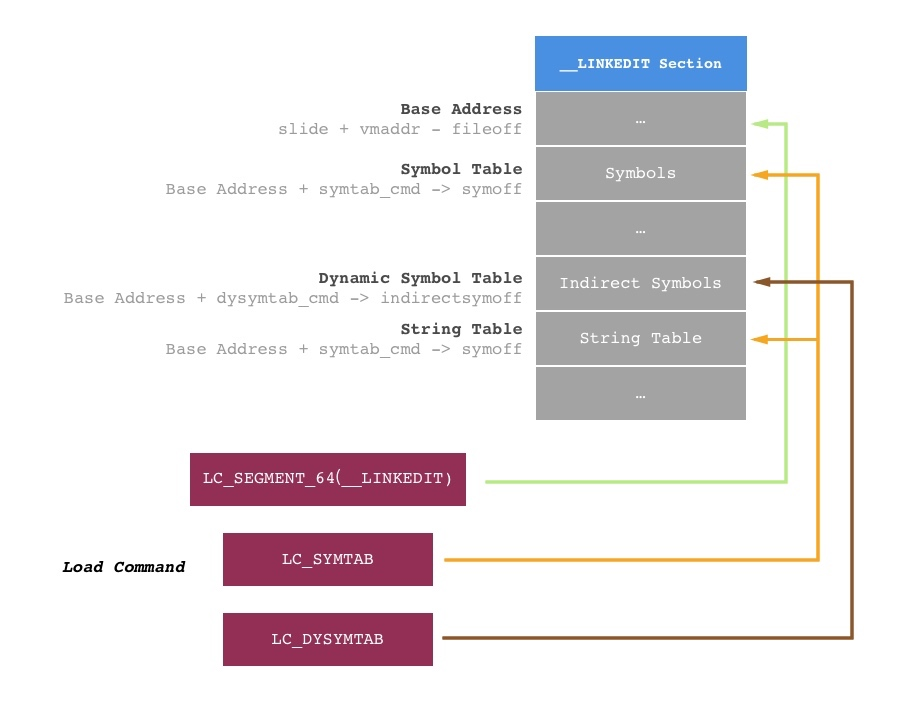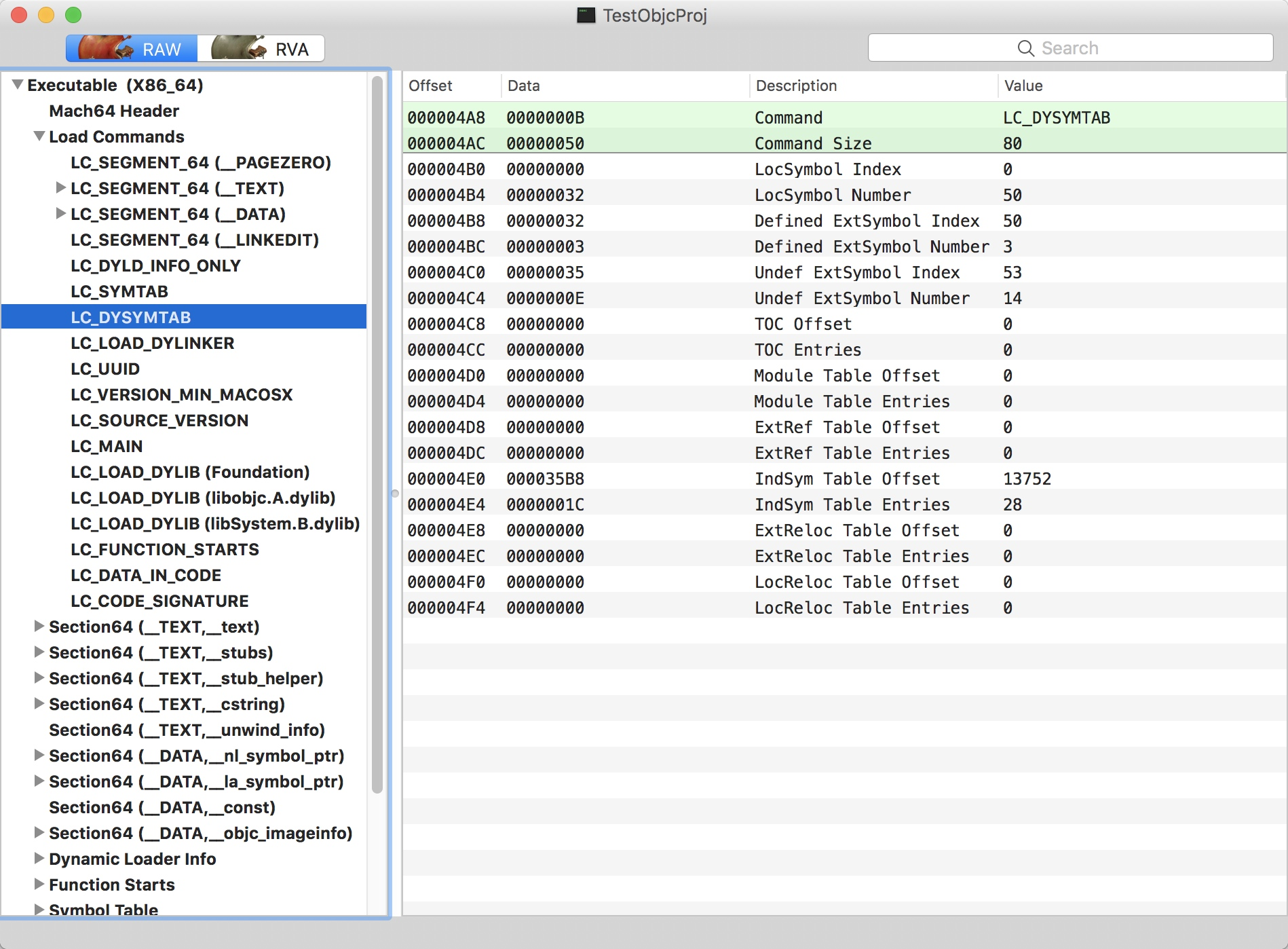$\begin{equation} \left\{ \begin{array}{l@{\;=\;}l} symbol\_offset=symtab\_cmd\to symoff=12680_{(oct)}=3188_{(hex)}\\ indirect\_symbol\_offset=dysymtab\_cmd\to symoff =14008_{(oct)}=35B8_{(hex)}\\ string\_table\_offset=symtab\_cmd\to stringoff=13864_{(oct)}=3628_{(hex)} \end{array} \right. \end{equation}$ $\begin{equation} \left\{ \begin{array}{l@{\;=\;}l} symbol\_base=base\_address+symbol\_offset=slide+100003188_{(hex)}\\ indirect\_symbol\_base=base\_address+indirect\_symbol\_offset=slide+1000035B8_{(hex)}\\ string\_table\_base=base\_address+string\_table\_offset=slide+100003628_{(hex)} \end{array} \right. \end{equation}$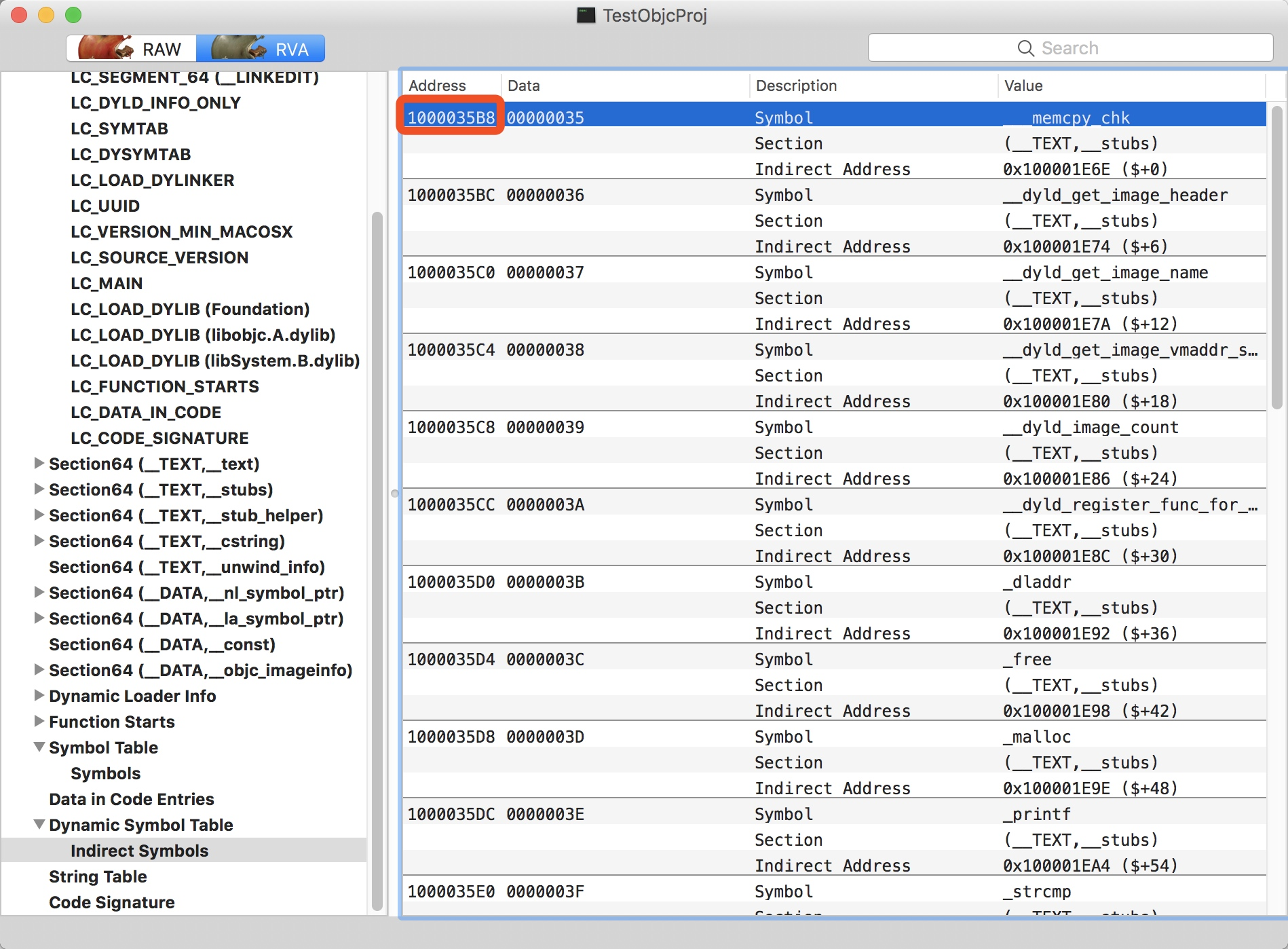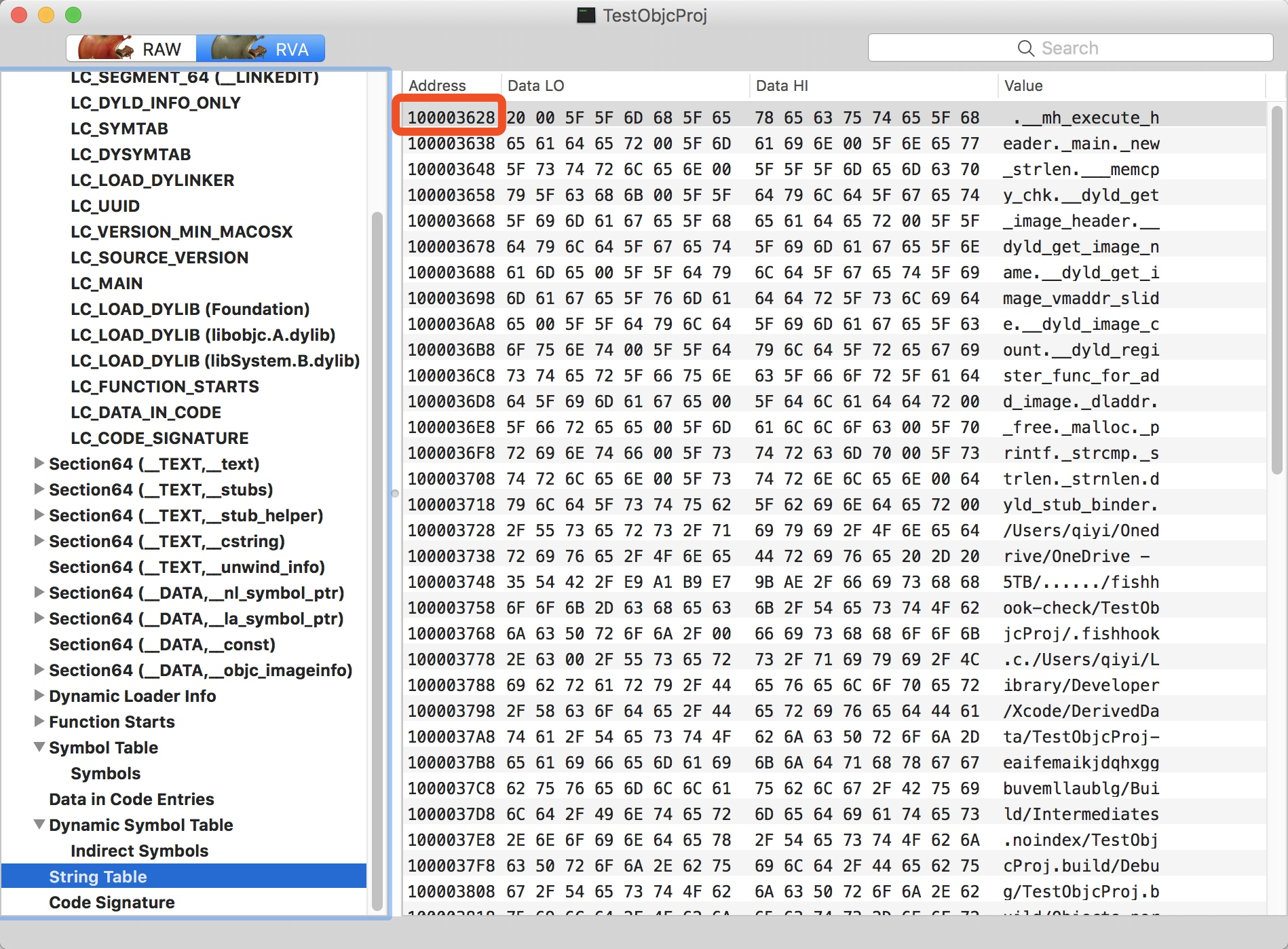## 跳转表 nl 和 la 绑定符号基址

$\begin{equation} \left\{ \begin{array}{l@{\;=\;}l} nl\_indirect\_sym\_index=13_{(oct)}\\ la\_indirect\_sym\_index=15_{(oct)}\\ \end{array} \right. \end{equation}$ $\begin{equation} \left\{ \begin{array}{l@{\;=\;}l} nl\_sym\_base\_addr&=nl\_indirect\_sym\_index\times size+indirect\_symbol\_base\\ &=13_{(oct)}\times 4 + (1000035B8_{(hex)}+slide)=1000035EC_{(hex)}+C\\ la\_sym\_base\_addr&=la\_indirect\_sym\_index\times size+indirect\_symbol\_base\\ &=15_{(oct)}\times 4 + (1000035B8_{(hex)}+slide)=1000035F4_{(hex)}+C\\ \end{array} \right. \end{equation}$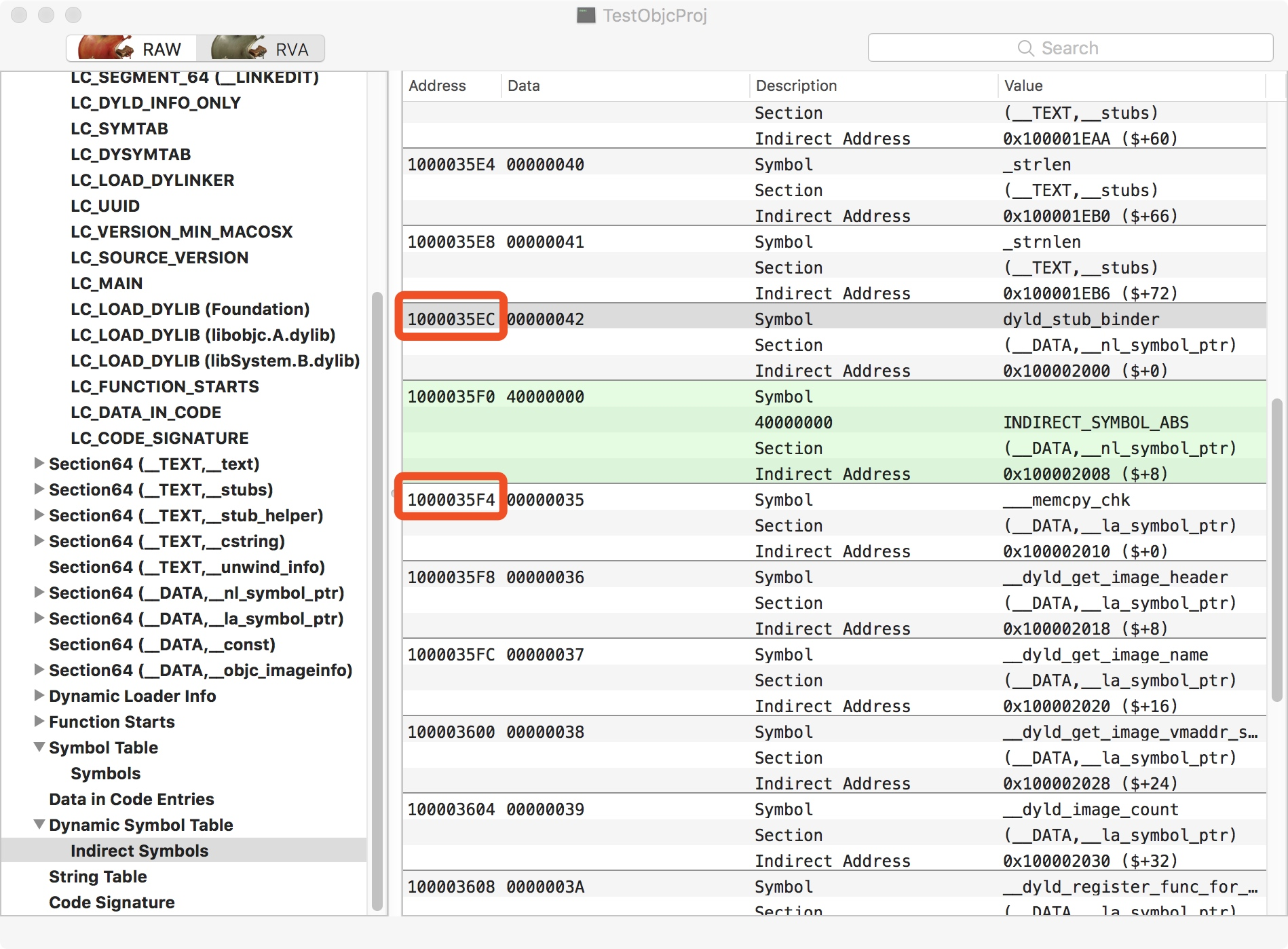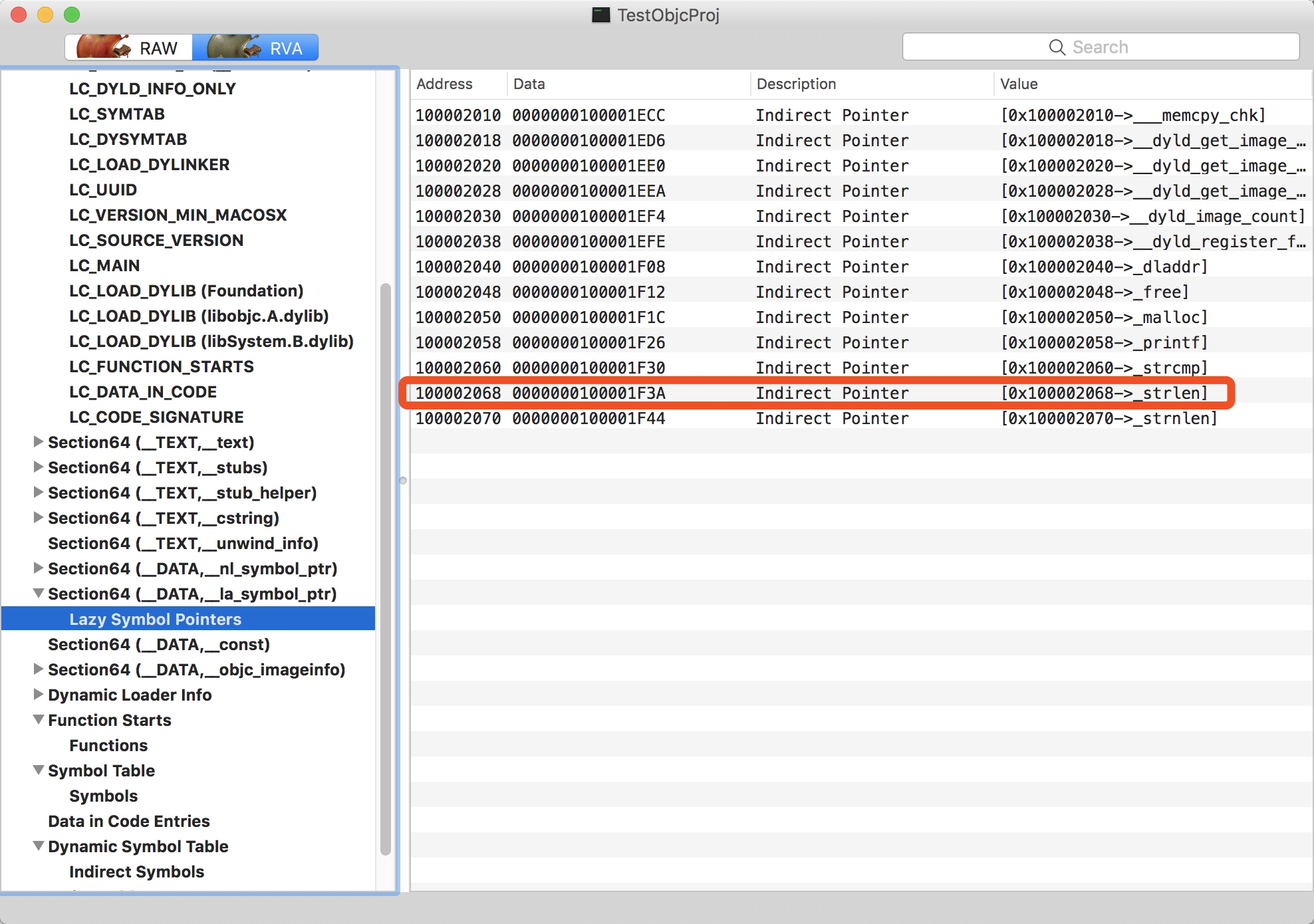## 在符号表中获取全部信息

Lazy Symbol Pointers 这里，我们最终获取到了 0x10002068 -> _strlen 这个值。它起始是对于当前 _strlen 方法在符号表中的一个映射，我们可以简单的理解为它就是在符号表中对应方法的下标

symtab[symtab_index].n_un.n_strx 从这个获取的方式来看，symtab_index 处的位置对应的是某个符号表的基址位，因此可以将类型转成 nlist_64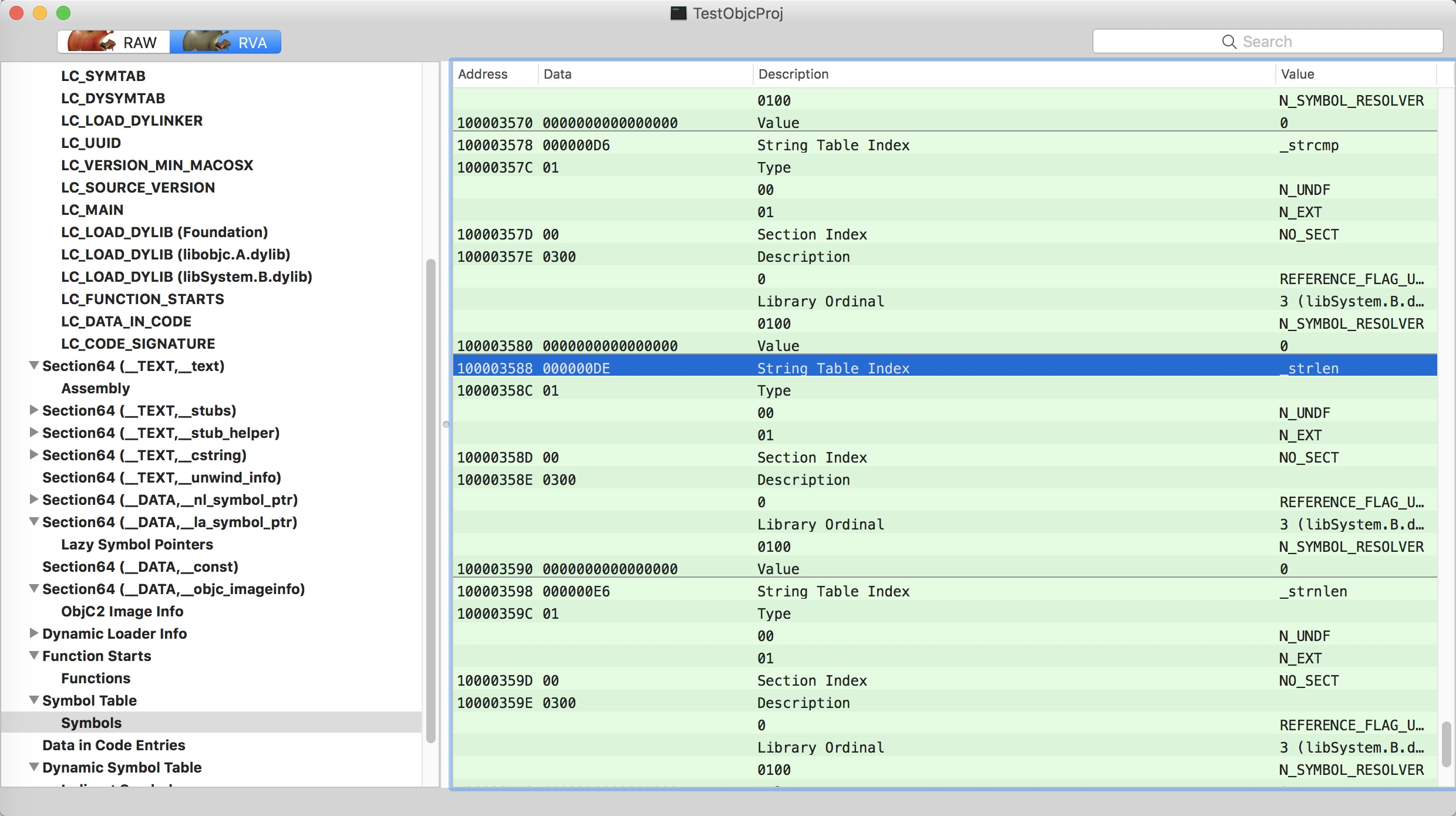0x1DA0 这个地址在原始的间接表指向的位置之前，所以我们大致断定它处在 _TEXT 段。并且__stub_helper, __cstring, __unwoind_info 这些 Session 是我们无法直接干预的位置，所以可以猜测 0x1DA0 落在我们的 __text Session 中。我们掏出 Hopper 来验证一下这个猜想，发现正是如此：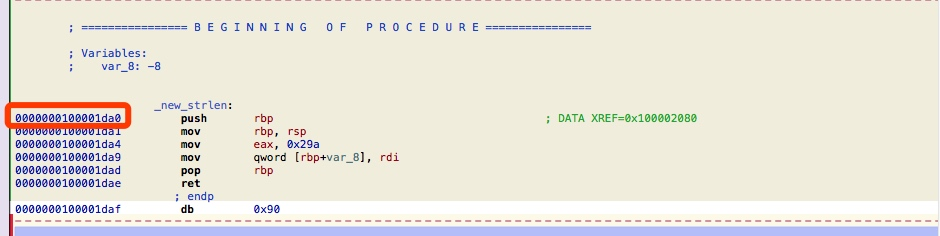## 尾声

fishhook 的原理探究至此就告一段落。通过这个源码探求，强化了对于 Mach-O 的学习和认识，也在之中学习到了 Facebook 对于 Hook C 方法这个很妙的技巧。最后不得不再惊叹一句，FB 真的是令技术人向往的地方。

## 参考